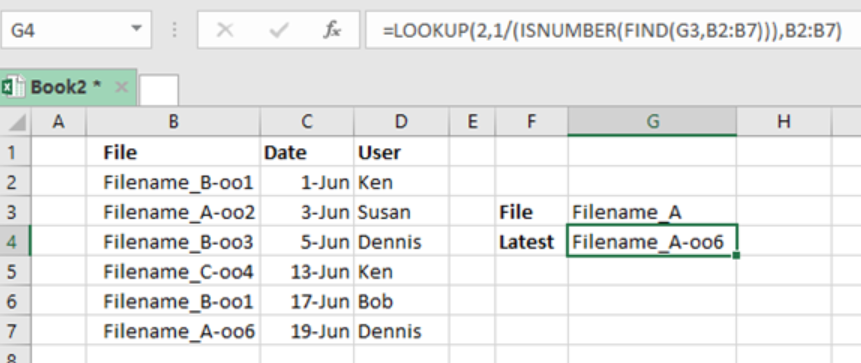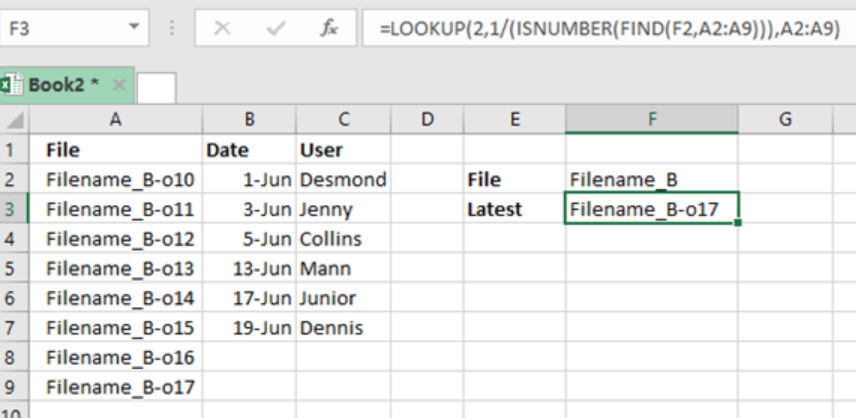Get instant live expert help with Excel or Google Sheets“My Excelchat expert helped me in less than 20 minutes, saving me what would have been 5 hours of work!”

#### Post your problem and you’ll get Expert help in seconds.

Your message must be at least 40 characters
Our professional Expert are available now. Your privacy is guaranteed.

# Lookup last file version

For us to lookup last file version in a list, we can use a formula which is based on a LOOKUP function together with ISNUMBER and FIND functions. This post provides an elaborative way of how one can lookup latest file version in excel.Figure 1: Using Lookup function to look up last file version

## Syntax of the formula

`=LOOKUP (2,1/(ISNUMBER(FIND(filename, range))), range)`

## Understanding how the formula works

•         Uses LOOKUP function to look for and retrieve last matching file name
•         LOOKUP value is 2
•         Lookup_vector is 1/(ISNUMBER(FIND(filename, range)))
•         The FIND function will look for  value in filename inside the range

The result for the FIND function looks like the one below;

`{1; #VALUE; 1;1; #VALUE!; #VALUE!;1}`

Here, 1 represents a match while #VALUE is an error representing non-matching file name. This goes to the ISNUMBER function and comes out as;

`{TRUE; FALSE; TRUE; FALSE; FALSE; TRUE}`

Note that the error value has been transformed to FALSE while the 1 becomes TRUE.

The array here is used as denominator with 1 as numerator. Result will look as the one below;

• `{1; #DIV/0!; 1;1; #DIV/0!; #DIV/0!;1}`

This goes into LOOKUP value as lookup_vector. It is important to mention that the LOOKUP function works in approximate match mode only, and ignore all error values.

• The LOOKUP function will thus look for 2, fail, then step back to previous number.

To further explain how this function works, let us consider the example below:

## ExampleFigure 2. Example of Lookup last file version

Step 2: Select the cell where you want the result to be displayed. It should be in front of cell labelled “Latest”.

Step 3: Input the formula `=LOOKUP (2,1/(ISNUMBER(FIND(F2,A2:A9))),A2:A9)`

Step 4: Press Enter

## Instant Connection to an Expert through our Excelchat Service

Most of the time, the problem you will need to solve will be more complex than a simple application of a formula or function. If you want to save hours of research and frustration, try our live Excelchat service! Our Excel Experts are available 24/7 to answer any Excel question you may have. We guarantee a connection within 30 seconds and a customized solution within 20 minutes.

### Did this post not answer your question? Get a solution from connecting with the expert.Another blog reader asked this question today on Excelchat:
Solution examplesVLOOKUP help. Trying to input data from database into new table
Solved by S. Q. in 40 minsI need to count number of dates from a timestamped date in column from the completed date column to show the number of days. I have not successfully been able to do this. I am a novice Excel user. Can you help?
Solved by O. B. in 15 minsUse the Vlookup Function to complete the "employee" column of table 2. Use "job Id" from table 2 as your lookup_value(s) and table 1 as your reference.
Solved by C. H. in 16 minsIf a cell in another sheet is populated I need a vlookup done. If the cell is not populated I need the cell to return blank.
Solved by T. D. in 60 minsI am trying to make a chart that turns a week range red if nothing is entered in the range. If something is entered then I would like it to turn green. Please Help
Solved by E. U. in 43 mins## Subscribe to Excelchat.coAnother blog reader asked this question today on Excelchat: